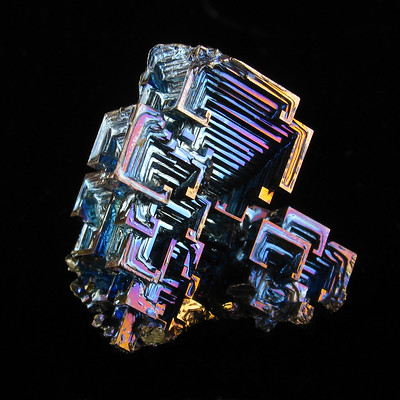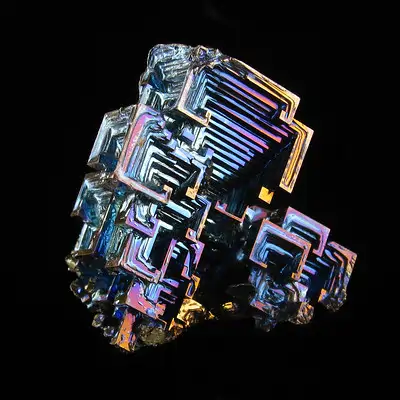# Perlin distortion in Rust

I remember seeing this post about using Perlin noise for image distortion a while back, and thought that it would be a good chance to play around with Rust, since manipulating images at the pixel level is where Python's relative slowness starts to show.To get our distorted image, for each (x, y) coordinate we generate some Perlin noise and get the pixel from (x + noise, y + noise) in the original image. The idea I had for making this look nice was to feed $sin$ functions into the Perlin noise function, cycling through the sin function a certain number of times in the $x$ and $y$ axes. In Rust that ended up looking like:

fn warp_image(
img: &RgbaImage,
x_freq: u32,
y_freq: u32,
distort_amount: f64,
warp_offset: f64,
) -> RgbaImage {
let perlin = Perlin::new();
let x_period = img.width() / x_freq;
let y_period = img.height() / y_freq;
let mut warped_frame = RgbaImage::new(img.width(), img.height());
for x in 0..img.width() {
for y in 0..img.height() {
let x_proportion: f64 = (x % x_period) as f64 / x_period as f64;
let x_amount = ((x_proportion + warp_offset) * 2_f64 * PI).sin();
let y_proportion: f64 = (y % y_period) as f64 / y_period as f64;
let y_amount = ((y_proportion + warp_offset) * 2_f64 * PI).sin();
let noise = perlin.get([x_amount, y_amount]);
let mut distorted_x = ((x as f64) + (noise * distort_amount)).round();
if distorted_x >= img.width() as f64 {
distorted_x = (img.width() - 1) as f64;
}
let mut distorted_y = ((y as f64) + (noise * distort_amount)).round();
if distorted_y >= img.height() as f64 {
distorted_y = (img.height() - 1) as f64;
}
let pixel = img.get_pixel(distorted_x as u32, distorted_y as u32);
warped_frame.put_pixel(x, y, pixel.clone());
}
}
warped_frame
}


There's an extra wrinkle in that code, the warp_offset, which also allowed me to cycle the noise over time. The end result is pretty nice:The speed of Rust is definitely nice here, the pixel manipulations (on a relatively small image) happen faster than 30x a second, without me having to think much about optimisation, although I'm not sure if I can hook this up to anything that could display the result in real time. The right way to do this kind of graphics processing is probably shaders, but alas, I am not a graphics programmer and don't know a lot about them.

You can check out the full code here!

Image credit: Bismuth crystal by Paul, CC BY-NC-SA 2.0